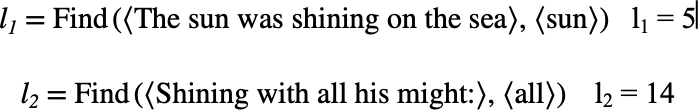# $$\text{Find}$$¶

You can use the $$\text{Find}$$ function to locate a sub-tuple within a tuple.

You can use the \find backslash command to insert this function.

The following variants of this function are available:

• $$\text{integer } \text{Find} \left ( \text{<tuple>}, \text{<sub>} \right )$$

• $$\text{integer } \text{Find} \left ( \text{<tuple>}, \text{<sub>}, \text{<starting at>} \right )$$

The $$\text{<tuple}$$ parameter is the tuple to be searched. The $$\text{<sub>}$$ is tht tuple containing entries to be found. The $$\text{<starting at>}$$ indicates the position to start searching from.

If the $$\text{<starting at>}$$ parameter is not supplied, the $$\text{Find}$$ will locate the first instance of $$\text{<sub>}$$ within $$\text{<tuple}$$. If the $$\text{<starting at>}$$ parameter is supplied, the not supplied, $$\text{Find}$$ will locate the first instance of $$\text{<sub>}$$ within $$\text{<tuple>}$$ at or after $$\text{<starting at>}$$.

Note that the $$\text{Find}$$ will trigger a run-time error or 0 if $$\text{<starting at>}$$ is not an integer value or is less than 1.

The $$\text{Find}$$ function will return the one-based index in $$\text{<tuple}$$ where $$\text{<sub>}$$ may be found. Zero (0) is returned if $$\text{<sub>}$$ could not be found.

Note

This function requires an exact match between tuple members. For this reason, this function may prove problematic with real or complex calculated values due to close or inexact results.

The Figure 138 shows how you can use the $$\text{Find}$$ function.Figure 138 Example Use Of The Find Function